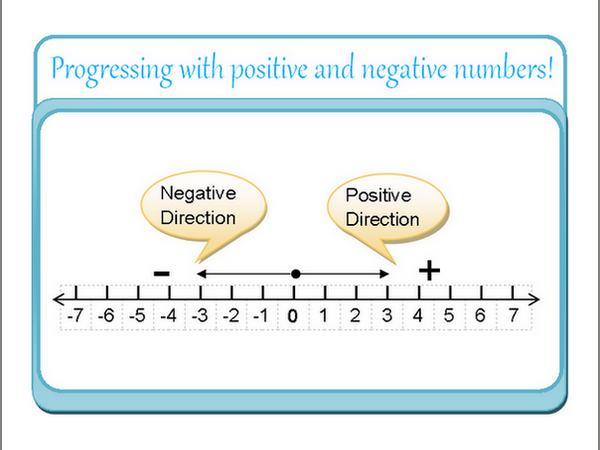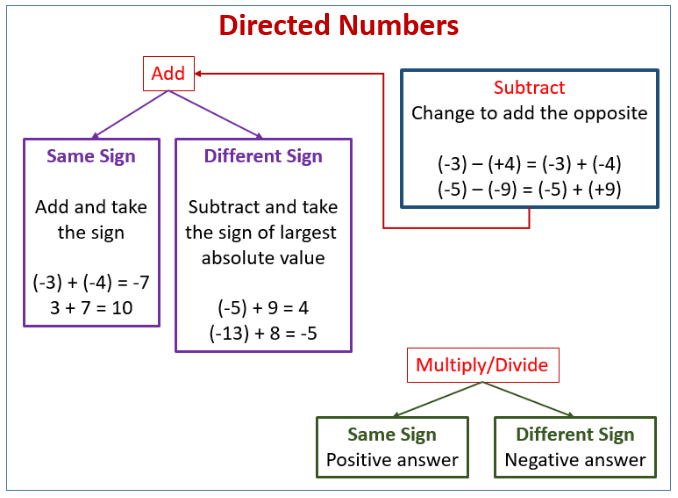# Multiplication and Division of Directed Numbers

Welcome to Class !!

In today’s Mathematics class, We will be discussing the Multiplication and Division of Directed Numbers. We hope you enjoy the class!

CONTENT

(i)    Addition and subtraction of directed numbers

(ii)   Multiplication of directed numbers

(iii)  Division of directed numbers

### ADDITION AND SUBTRACTION OF DIRECTED NUMBERSDirected numbers are the positive and negative numbers in any given number line e.g.

-4         -3         -2         -1         0          1          2          3           4

The (+) and (-) signs show the direction from the origin (o). To add a positive number move to the right on the number line

-2    -1         0          +1       +2       +3       +4

To subtract a positive number, move to the left on the number line

Subtraction

-2    -1         0          +1       +2       +3       +4

Worked examples

Simplify the following

1. (-2_+(-4)
2. (-6)+(+6)+0
3. 6-(-3)-(-4)

Solution

• (-2)+(-4)=2+4=-6

-6         -5         -4         -3         -2         -1         0          +1       +2

• (-6)+(-6)+0= 6+6+0 =- 0

-6         -5         -4         -3         -2         -1         0          +1       +2       +3

• -(-3)-(-4)= 6+3+4 =- 13

-1         0          +1       +2   +3    +4   +5   +6   +7    +8   +9    +10   +11    +12    +13

SELF EVALUATION

Simplify the following

1. (+6)-(+10)
2. 12-(+3)-8
3. (-5)+(-5)+(-5)
4. (-8)-(-2)+(-2)

##### MULTIPLICATION OF DIRECTED NUMBERS

Multiplication is a short way of writing repeated addition e.g. 3×4=4+4+4 =12.

When directed numbers are multiplied together, two like signs give a positive result, while two unlike signs give a negative result in general

(i) (+a) x (-b) =+ab

(ii) (-a) x (-b) =+ab

(iii) (+a) x(-b)= -ab

(iv) (-a) x (+b) =-ab

Worked examples

Simplify the following

(a) (+1/2) x (+1/4) = +1/8

(b) (+17) x (-3) = -51

(c ) (- 91/3) x (+2/5) = -56/15 = -311/15

DIVISION OF DIRECTED NUMBERS

The rules of multiplication of directed numbers also apply to the division of directed numbers

1. (+a) ÷ (+b)= +(a/b)
2. (-a) ÷ (-b) = +(a/b)
3. (+a) ÷ (-b) = -(a/b)
4. (-a) ÷ (+b) = -(a/b)

Classwork

1. Simplify the following
• (-36) ÷ (+4)
• (-4) ÷(-12)
• (-6) x (-5)
• -10 x 3

1. Complete the following
• (+6) + (5) =
• (+6) + (9) =
• (-6) + (=5) =
• (+7) + (=4) =
• (+8) – (+6) =WEEKEND ASSIGNMENT

1. Simplify 12 – (+3) – 8= ____ a) -1 (b) +1 (c)-2 (d)+2
2. Simplify (-3) – (-1)= ____ a) -2 (b) -1 (c)+1 (d) +2
3. Simplify (+15) x (-4)= ____ a) -20 (b) -60 (c)+20 (d) +60
4. Divide -18 by -3 = ____ a) -6 (b) +6 (c)-21 (d) +15
5. Divide -5 by -15 = ____ a) +1/3 (b) -1/3 (c) +1/5 (d) -1/5

THEORY

1      a). Simplify 4/9 of (-2 4)

b) Simplify (-2) + (-7) using number lines.

2    Simplify

1. a) 7 x (-6.2)
2. b)   -112 ÷ -4

We have come to the end of this class. We do hope you enjoyed the class?

Should you have any further question, feel free to ask in the comment section below and trust us to respond as soon as possible.

In our next class, we will be talking about Algebraic Expressions. We are very much eager to meet you there.

Are you a Parent? Share your quick opinion and win free 2-month Premium Subscription

Don`t copy text!